# Difference between revisions of "Mathematical expectation"

mean value, of a random variable

2010 Mathematics Subject Classification: Primary: 60-01 [MSN][ZBL]

A numerical characteristic of the probability distribution of a random variable. In the most general setting, the mathematical expectation of a random variable,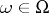, is defined as the Lebesgue integral with respect to a probability measure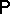on a given probability space: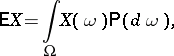(*)

provided the integral exists. The mathematical expectation of a real-valued random variable may be calculated also as the Lebesgue integral ofwith respect to the probability distribution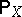of: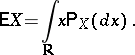The mathematical expectation of a function inis expressible in terms of the distribution; for example, ifis a random variable with values inandis a single-valued Borel function of, thenIf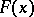is the distribution function of, then the mathematical expectation ofcan be represented as the Lebesgue–Stieltjes (or Riemann–Stieltjes) integral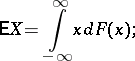here integrability ofin the sense of (*) is equivalent to the finiteness of the integral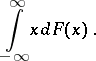In particular cases, ifhas a discrete distribution with possible values,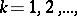and corresponding probabilities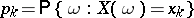, then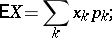ifhas an absolutely continuous distribution with probability density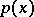, then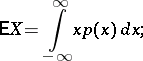moreover, the existence of the mathematical expectation is equivalent to the absolute convergence of the corresponding series or integral.

Main properties of the mathematical expectation:

a)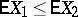whenever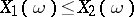for all;

b)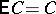for every real constant;

c)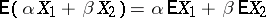for all realand;

d)if the series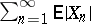converges;

e)for convex functions;

f) every bounded random variable has a finite mathematical expectation;

g)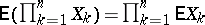if the random variablesare mutually independent.

One can naturally define the notion of a random variable with an infinite mathematical expectation. A typical example is provided by the return times in certain random walks (see, e.g., Bernoulli random walk).

The mathematical expectation is used to define many numerical functional characteristics of probability distributions (as the mathematical expectations of appropriate functions in the given random variables), for example, the generating function, the characteristic function and the moments (cf. Moment) of all orders, in particular, the variance (cf. Dispersion) and the covariance.

The mathematical expectation is a characteristic of the location of the values of a random variable (the mean value of its distribution). Here, the mathematical expectation serves as a "typical" value from the distribution and its role is analogous to the role played in mechanics by the statical momentum — the coordinates of the barycentre of a mass distribution. The mathematical expectation differs from other characteristics of location which describe the distribution in general terms — like the median (cf. Median (in statistics)) and the mode, by the higher importance that it and its corresponding scatter characteristic, the variance, have in limit theorems of probability theory. The meaning of the mathematical expectation is most completely revealed by the law of large numbers (see also Chebyshev inequality in probability theory) and the strong law of large numbers. In particular, ifis a sequence of mutually-independent identically-distributed random variables with finite mathematical expectation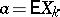, then, asand for every,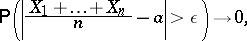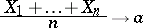The notion of the mathematical expectation as the expected value of a random variable was first noticed in the 18th century in connection with the theory of games of chance. Initially the term "mathematical expectation" was introduced as the expected pay-off of a player, equal to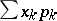for possible pay-offswith respective probabilities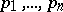. Primary contributions in the generalization and utilization of the notion of the mathematical expectation in its contemporary meaning are due to P.L. Chebyshev.